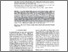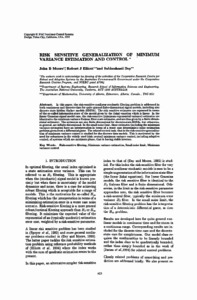# Risk Sensitive Generalization of Minimum Variance Estimation and Control

Moore, John B and Elliott, Robert J and Dey, Subhrakanti (1995) Risk Sensitive Generalization of Minimum Variance Estimation and Control. IFAC Proceedings Volumes, 28 (14). pp. 423-428. ISSN 1474-6670Previewmore...Add this article to your Mendeley library

## Abstract

In this paper, the risk-sensitive nonlinear stochastic filtering problem is addressed in both continuous and discrete-time for quite general finite-dimensional signal models, including also discrete state hidden Markov models (HMMs). The risk sensitive estimates are expressed in terms of the so-called information state of the model given by the Zakai equation which is linear. In the linear Gaussian signal model case, the risk-sensitive (minimum exponential variance) estimates are identical to the minimum variance Kalman filter state estimates, and are thus given by a finite dimensional estimator. The estimates are also finite dimensional for discrete-state HMMs, but otherwise, in general, axe infinite dimensional. In the small noise limit, these estimates (including the minimum variance estimates) have an interpretation in terms of a worst case deterministic noise estimation problem given from a differential game. The related control task, that is the risk-sensitive generalization of minimum-variance control is studied for the discrete-time models. This is motivated by the need for robustness in the widely used (risk neutral) minimum variance control, including adaptive control, of systems which are minimum phase, that is having stable inverses.

Item Type: Article Risk-sensitive filtering; Minimum variance estimation; Small noise limit; Minimum variance control; Faculty of Science and Engineering > Electronic Engineering 12733 https://doi.org/10.1016/S1474-6670(17)46866-2 Subhrakanti Dey 09 Apr 2020 10:18 IFAC Proceedings Volumes Elsevier YesItem control page